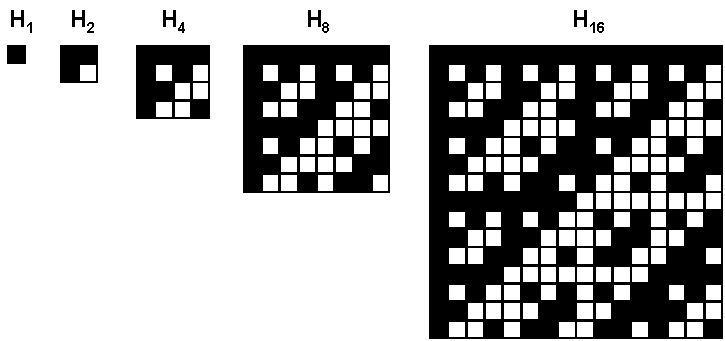COS 126Pictures From Space Programming Assignment xyz Due: Wednesday, 11:59pm

How are pictures sent from the Mariner spacecraft back to earth? The spacecraft records the image internally as a sequence of 0's and 1's, and then sends the information back to earth. Even at the speed of light, the data may take several minutes to reach earth, and during its journey the signal gets somewhat garbled. By building redundancy into the signal, scientists back on earth can recover the original image free of any errors. Error correcting codes are one technique for building in the appropriate redundancy.

Your goal is to write a program to correct errors in a transmission using the Hadamard error correction code. Each pixel of the image is represented by one of 32 possible gray-scale levels from 0 (white) to 31 (black). Later in the assignment, we'll deal with the case where each pixel is represented by three RGB color values, each in the range from 0 to 255. We could represent each pixel in binary, using 5 bits. For example, if the spacecraft observed the gray-scale level of a pixel to be 13, it could send the signal:

`01101.`
Instead, for reasons that will become clear, we'll use the less parsimonious 32 bit encoding:
`10100101010110101010010101011010.`
When the pattern arrives at the telemetry receiving station on earth, the bit pattern might have been mangled to:
```10000101010101101010000001011010
*         **       * *```
where the asterisks denote errors that have occurred during transmission. Because we encode each gray scale value with 32 bits instead of 5, there is some hope of identifying the errors and recovering the original message intact. A little bit of mathematical magic is needed.

Hadamard code.  The N x N Hadamard matrix is an N x N table of 0's and 1's with a very special property: every two rows differ in exactly N / 2 places. The Hadamard matrices for N = 1, 2, 4, 8, 16, and N = 32 are shown below, where white represents 0 and black represents 1. Note that row 13 (start counting from 0 as usual) of the order 32 Hadamard matrix is

`10100101010110101010010101011010`
which explains why we chose that encoding for gray-scale level 13 above. In general, the 2N x 2N Hadamard matrix is constructed by aligning 4 copies of the N x N Hadamard matrix in the form of a large square, and then inverting all of the entries in the lower right N x N copy.Now, when a 32 bit codeword is received on earth, it is compared with each of the rows of the 32 x 32 Hadamard matrix until a "match" is found. If no errors were made in transmission, then the codeword will perfectly match (exactly) one of the rows. Otherwise, we choose the row in the Hadamard matrix that it most closely resembles, i.e., the number of bits the two have in common. If there was one error made in transmission, then the codeword will differ from one of the Hadamard rows in exactly one place. It will differ from every other row in at least 15 places, so there is no mistaking a match! Why? The Hadamard property guarantees that every two rows of the matrix differ in at least 16 places, so if the codeword is one bit away from one row, it must be 15 (or more) away from every other row. A similar thing happens if there are two, three, or even seven errors. However, if 8 errors occur, then the codeword may be 8 places away from an "incorrect" row.

Extra credit.

Challenge for the bored.  NSSDC Pictures

This assignment was created by Kevin Wayne, and was inspired by Excursion 12 in The New Turing Omnibus.